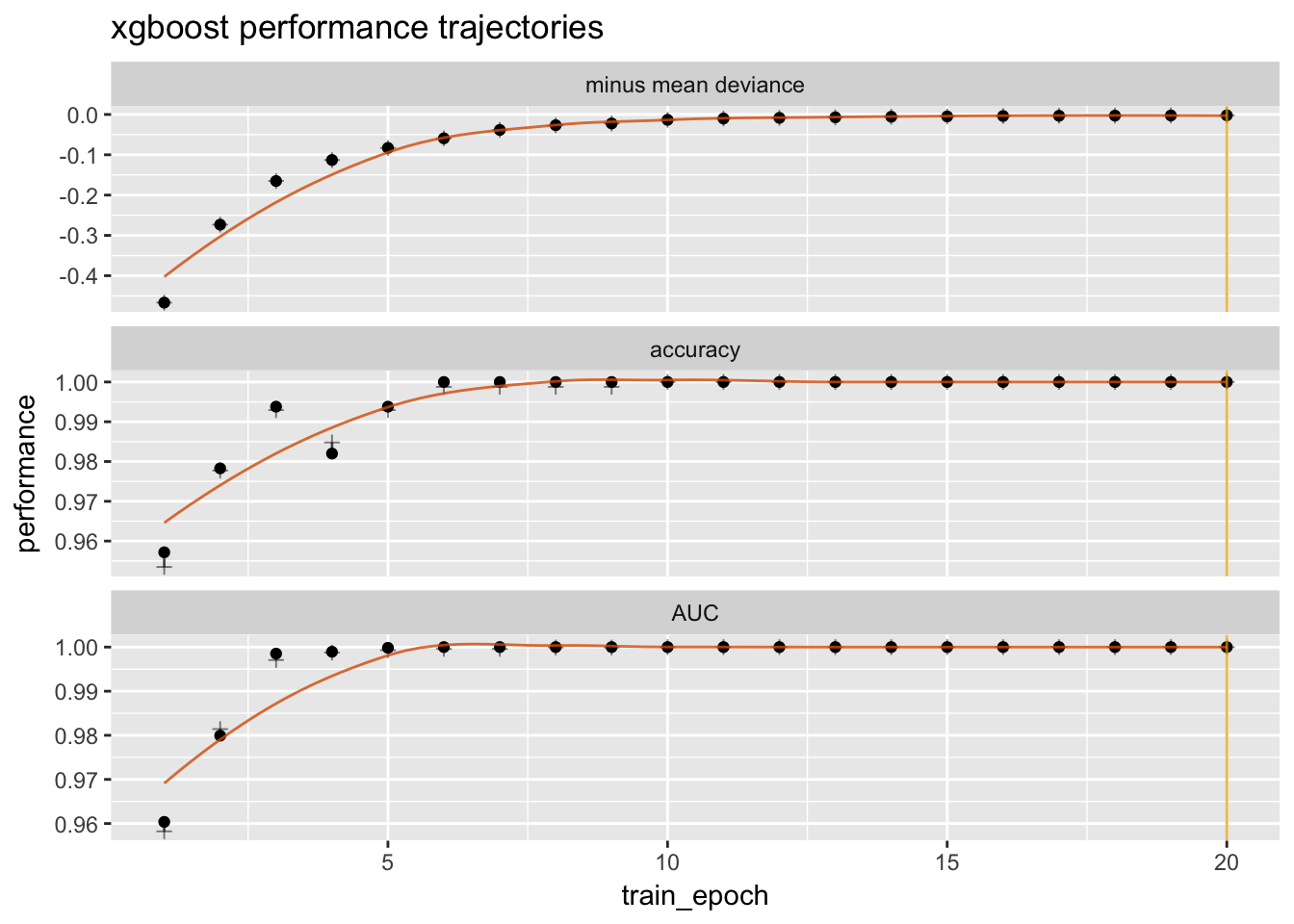# Plotting xgboost Performance Trajectories

#### 2018-01-01

Below is the standard performance trajectory history from xgboost.

library("xgboost")
library("WVPlots")
library("seplyr")
library("sigr")
library("kableExtra")
options(knitr.table.format = "html")

# From:
data(agaricus.train,
package='xgboost')
data(agaricus.test,
package='xgboost')
train <- agaricus.train
test <- agaricus.test
epochs <- 20

bstSparse <-
xgboost(data = train$data, label = train$label,
max.depth = 2,
eta = 1,
nround = epochs,
objective = "binary:logistic")
##   train-error:0.046522
##   train-error:0.022263
##   train-error:0.007063
##   train-error:0.015200
##   train-error:0.007063
##   train-error:0.001228
##   train-error:0.001228
##   train-error:0.001228
##   train-error:0.001228
##  train-error:0.000000
##  train-error:0.000000
##  train-error:0.000000
##  train-error:0.000000
##  train-error:0.000000
##  train-error:0.000000
##  train-error:0.000000
##  train-error:0.000000
##  train-error:0.000000
##  train-error:0.000000
##  train-error:0.000000
head(bstSparse$evaluation_log) ## iter train_error ## 1: 1 0.046522 ## 2: 2 0.022263 ## 3: 3 0.007063 ## 4: 4 0.015200 ## 5: 5 0.007063 ## 6: 6 0.001228 Next we re-evaluate the model performance trajectory both on training and test data using metrics of our own choosing. score_model <- function(model, epoch, data, datasetname) { pred <- predict(model, newdata = data$data,
ntreelimit = epoch)
acc <- mean(data$label == ifelse(pred>=0.5, 1.0, 0.0)) dev <- sigr::calcDeviance(pred, ifelse(data$label>=0.5,
TRUE,
FALSE))
auc <- sigr::calcAUC(pred,
ifelse(data$label>=0.5, TRUE, FALSE)) data.frame(dataset = datasetname, epoch = epoch, accuracy = acc, mean_deviance = dev/nrow(data$data),
AUC = auc,
stringsAsFactors = FALSE)
}

score_model_trajectory <- function(model,
epochs,
data,
datasetname) {
evals <- lapply(epochs,
function(epoch) {
score_model(model,
epoch,
data,
datasetname)
})
r <- dplyr::bind_rows(evals)
colnames(r) <- paste(datasetname,
colnames(r),
sep = "_")
r
}

eval <-
cbind(
score_model_trajectory(bstSparse,
seq_len(epochs),
train,
"train"),
score_model_trajectory(bstSparse,
seq_len(epochs),
test,
"test"))
cols <- c("train_epoch", "train_accuracy",
"train_mean_deviance", "train_AUC",
"test_accuracy", "test_mean_deviance",
"test_AUC")
eval <- eval[, cols, drop = FALSE]
knitr::kable(head(eval))
train_epoch train_accuracy train_mean_deviance train_AUC test_accuracy test_mean_deviance test_AUC
1 0.9534777 0.4667512 0.9582280 0.9571695 0.4533720 0.9603733
2 0.9777368 0.2733163 0.9814132 0.9782744 0.2757484 0.9799301
3 0.9929372 0.1650616 0.9970700 0.9937927 0.1609212 0.9985184
4 0.9847996 0.1129484 0.9987570 0.9819988 0.1166577 0.9989428
5 0.9929372 0.0830270 0.9992985 0.9937927 0.0765738 0.9998302
6 0.9987717 0.0592123 0.9995853 1.0000000 0.0532618 1.0000000

At this point we have gotten to the very wide table one might expect to have on hand from a training procedure. So only the code this point and below is actually the plotting procedure.

We can then plot the performance trajectory using WVPlots::plot_fit_trajectory() plot.

cT <- dplyr::tribble(
~measure,                 ~training,             ~validation,
"minus mean deviance",    "train_mean_deviance", "train_mean_deviance",
"accuracy",               "train_accuracy",      "test_accuracy",
"AUC",                    "train_AUC",           "test_AUC"
)
cT %.>%
knitr::kable(.) %.>%
kable_styling(., full_width = F) %.>%
column_spec(., 2:3, background = "yellow")
measure training validation
minus mean deviance train_mean_deviance train_mean_deviance
accuracy train_accuracy test_accuracy
AUC train_AUC test_AUC
plot_fit_trajectory(eval,
column_description = cT,
epoch_name = "train_epoch",
needs_flip = "minus mean deviance",
pick_metric = "minus mean deviance",
title = "xgboost performance trajectories")
## Warning: 'moveValuesToRowsD' is deprecated.
## See help("Deprecated")• An optional additional training over-fit penalized dashed curve is added. The default is: this is the validation score minus 10% of the excess generalization error (the difference in training and validation performance).